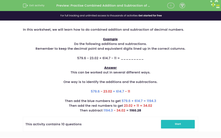# Practise Combined Addition and Subtraction of Decimals

In this worksheet, students will work out combined calculations involving addition and subtraction of decimals.Key stage:  KS 3

Curriculum topic:   Number

Curriculum subtopic:   Use Four Operations for All Numbers

Difficulty level:#### Worksheet Overview

In this activity, we will learn how to do combined addition and subtraction of decimal numbers.

Remember to keep the decimal point and equivalent digits lined up in the correct columns.

Any gaps that are left in the calculation should be filled in with zeros.

For example:

36.5 - 4.742

We need to write the two numbers so that the decimal points are lined up, and then fill in the gaps with zeros.

We can then do the sum as a normal subtraction sum. Make sure that you line up the decimal point correctly in the answer!

36.500 -

04.742

---------------

31.758

Sometimes, we have more complicated calculations to do that involve both addition and subtraction.

Example

579.6 - 23.02 + 614.7 - 11 = _________

This can be worked out in several different ways.

One way is to identify the additions and the subtractions.

579.6 - 23.02 + 614.7 - 11

Then add the blue numbers to get 579.6 + 614.7 = 1194.3

Then add the red numbers to get 23.02 + 11 = 34.02

Then subtract 1194.3 - 34.02 = 1160.28

Let's have a go at some questions.

### What is EdPlace?

We're your National Curriculum aligned online education content provider helping each child succeed in English, maths and science from year 1 to GCSE. With an EdPlace account you’ll be able to track and measure progress, helping each child achieve their best. We build confidence and attainment by personalising each child’s learning at a level that suits them.

Get started••••